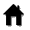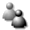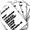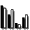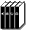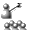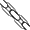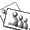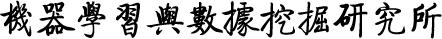Search
»

# MOMS

Description: This package includes the JAVA code of the MOMS algorithm  for maximizing monotone k-submodular functions under a size constraint. It uses a simple multi-objective evolutionary algorithm combined with randomized local search to solve the bi-objective reformulation of the original problem: maximizing the monotone k-submodular objective function and minimizing the size. A Readme file and an example file are included in the package. In the 'Example.java', you will find an example of using this code for the application of sensor placement with k kinds of sensors on a real-world data set (http://db.csail.mit.edu/labdata/labdata.html).

Reference:

 Chao Qian, Jing-Cheng Shi, Ke Tang, and Zhi-Hua Zhou. Constrained Monotone k-Submodular Function Maximization Using Multi-objective Evolutionary Algorithms with Theoretical Guarantee. IEEE Transactions on Evolutionary Computation.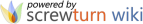© LAMDA, 2022